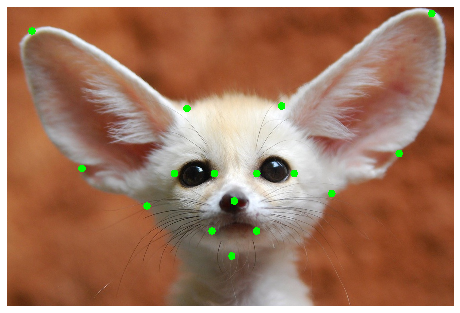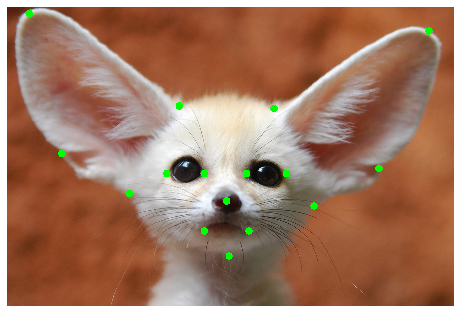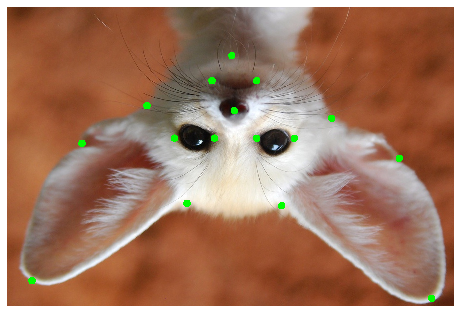# Using Albumentations to augment keypoints¶

In this notebook we will show how to apply Albumentations to the keypoint augmentation problem. Please refer to A list of transforms and their supported targets to see which spatial-level augmentations support keypoints. You can use any pixel-level augmentation to an image with keypoints because pixel-level augmentations don't affect keypoints.

Note: by default, augmentations that work with keypoints don't change keypoints' labels after transformation. If keypoints' labels are side-specific, that may pose a problem. For example, if you have a keypoint named `left arm` and apply a HorizontalFlip augmentation, you will get a keypoint with the same `left arm` label, but it will now look like a `right arm` keypoint. See a picture at the end of this article for a visual example.

If you work with such type of keypoints, consider using SymmetricKeypoints augmentations from albumentations-experimental that are created precisely to handle that case.

### Import the required libraries¶

``````import random

import cv2
from matplotlib import pyplot as plt

import albumentations as A
``````

### Define a function to visualize keypoints on an image¶

``````KEYPOINT_COLOR = (0, 255, 0) # Green

def vis_keypoints(image, keypoints, color=KEYPOINT_COLOR, diameter=15):
image = image.copy()

for (x, y) in keypoints:
cv2.circle(image, (int(x), int(y)), diameter, (0, 255, 0), -1)

plt.figure(figsize=(8, 8))
plt.axis('off')
plt.imshow(image)
``````

#### Get an image and annotations for it¶

``````image = cv2.imread('images/keypoints_image.jpg')
image = cv2.cvtColor(image, cv2.COLOR_BGR2RGB)
``````

#### Define keypoints¶

We will use the `xy` format for keypoints' coordinates. Each keypoint is defined with two coordinates, `x` is the position on the x-axis, and `y` is the position on the y-axis. Please refer to this article with the detailed description of formats for keypoints' coordinates - https://albumentations.ai/docs/getting_started/keypoints_augmentation/

``````keypoints = [
(100, 100),
(720, 410),
(1100, 400),
(1700, 30),
(300, 650),
(1570, 590),
(560, 800),
(1300, 750),
(900, 1000),
(910, 780),
(670, 670),
(830, 670),
(1000, 670),
(1150, 670),
(820, 900),
(1000, 900),
]
``````

### Visualize the original image with keypoints¶

``````vis_keypoints(image, keypoints)
``````### Define a simple augmentation pipeline¶

``````transform = A.Compose(
[A.HorizontalFlip(p=1)],
keypoint_params=A.KeypointParams(format='xy')
)
transformed = transform(image=image, keypoints=keypoints)
vis_keypoints(transformed['image'], transformed['keypoints'])
``````### A few more examples of augmentation pipelines¶

``````transform = A.Compose(
[A.VerticalFlip(p=1)],
keypoint_params=A.KeypointParams(format='xy')
)
transformed = transform(image=image, keypoints=keypoints)
vis_keypoints(transformed['image'], transformed['keypoints'])
``````We fix the random seed for visualization purposes, so the augmentation will always produce the same result. In a real computer vision pipeline, you shouldn't fix the random seed before applying a transform to the image because, in that case, the pipeline will always output the same image. The purpose of image augmentation is to use different transformations each time.

``````random.seed(7)
transform = A.Compose(
[A.RandomCrop(width=768, height=768, p=1)],
keypoint_params=A.KeypointParams(format='xy')
)
transformed = transform(image=image, keypoints=keypoints)
vis_keypoints(transformed['image'], transformed['keypoints'])
``````